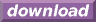#Calcute: freeware calculator

## Cosecant (csc)

The cosecant of an angle is defined using the right-triangle formed by this angle: it is the ratio of the triangle's hypotenuse to its opposite side. Calcute implements it with the csc function.

```csc(1)
1.18839510578
```

In the above example, the given angle is in radians.

The triangle involved in the definition of the cosecant function is commonly shown in the cartesian plane with its adjacent side on the X axis and its angle point located at the origin (0,0). The other end of the triangle's hypotenuse marks a point on the periphery of a circle centered at the origin; the circle's radius is the length of the hypotenuse. If the angle is increased from 0 to 2 pi radians (0 to 360 degrees) then the end point of the hypotenuse traces a full circle. As the angle of the hypotenuse changes, so does its cosecant value as the shape of the corresponding triangle changes to match.

The cosecant is undefined at multiples of 1/2 of a full circle (including zero). At these angles, the triangle geometrically vanishes into a simple line segment on the X axis. The value of the triangle's opposite side is zero and division by zero is undefined. For example, csc is undefined at pi radian, or equivalently at 180 degrees or at 200 gradiens.

The cosecant function is periodic. Except for those angles that represent multiples of 1/2 of a circle, it is defined for all real numbers. As angle values increase (or decrease) outside the range of [0, 2 pi] radians that represent one full rotation, the value of csc for these angles repeats itself periodically with each new rotation.